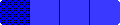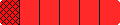Home
 Teaching Students Fraction Concepts Grades 3 to 4 .The six files below provide an introduction to grades 3-4 students in the use of the Fraction Bars model for illustrating the part-to-whole concept of a fraction equality inequality addtion subtraction and multiplication of a fraction by a fraction.Usually instruction is centered around teaching rules for using fractions but the purpose of the illustrations in the following files is to suggest ways to provide an intuitive understanding of fraction notation and fraction relations. These illustrations will help show teachers how to make connections between models for fractions and learning about fractions. Introduction to Fraction Bars 5-Bars and Fraction Bingo (equality) Small Step Race (equality) Capture (subtraction) Fractions and Operations Linear Model - Number Lines The six files above contain comments and suggestions for teachers as well as tables from NCTM's Standards and NAEP's research results which document some of the common difficulties students experience in learning about fractions. Students may find such tables interesting. The file Overhead Masters for Workshop can be used to make transparencies of the selected games and activities in these six files. Transparent Fraction Bars are recommended for illustrating the games and activities. These can be ordered or produced from the abbreviated set of Fraction Bars masters. For further teaching suggestions and complete day-by-day lessons see the Grade 3-4 Fraction Bars Teacher's Guide and for activity sheets and tests see Grades 3-4 Fraction Bars Teacher Resource Package.
HomeHome